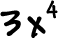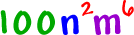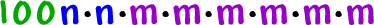What if x = -2?  Be careful!TRY IT:

Write out whatmeans:

Let x = 2 and simplify:

Let x = -5 and simplify:

Here's something a little messier:

What does this thing mean?The number hangs around in front... and we can expand out those exponents:So, why do we have exponents?

Look at this thing!